# For many technical applications, it is desirable to have a uniform magnetic field, i.e. a field...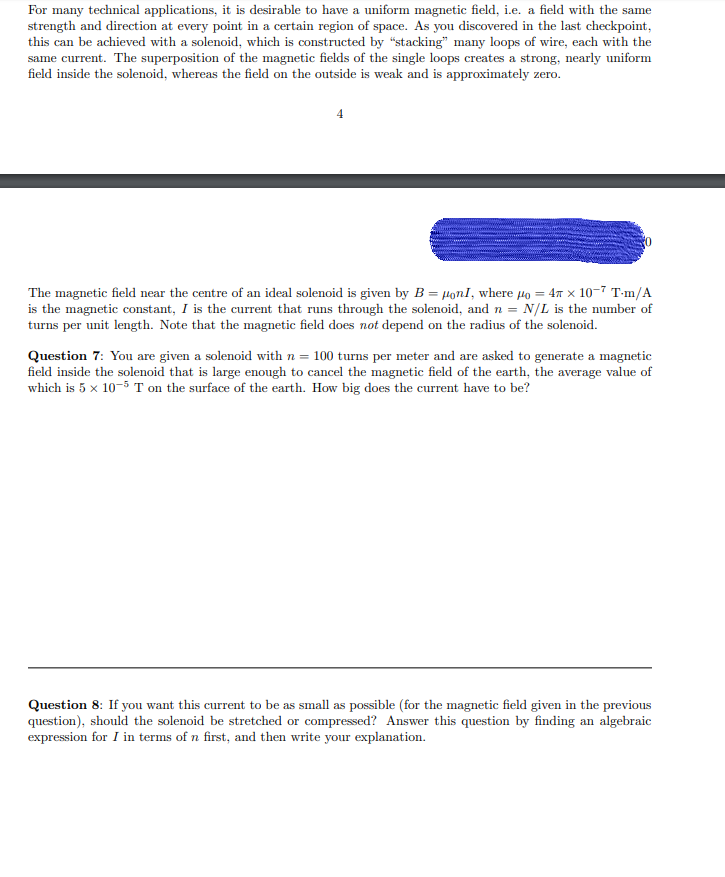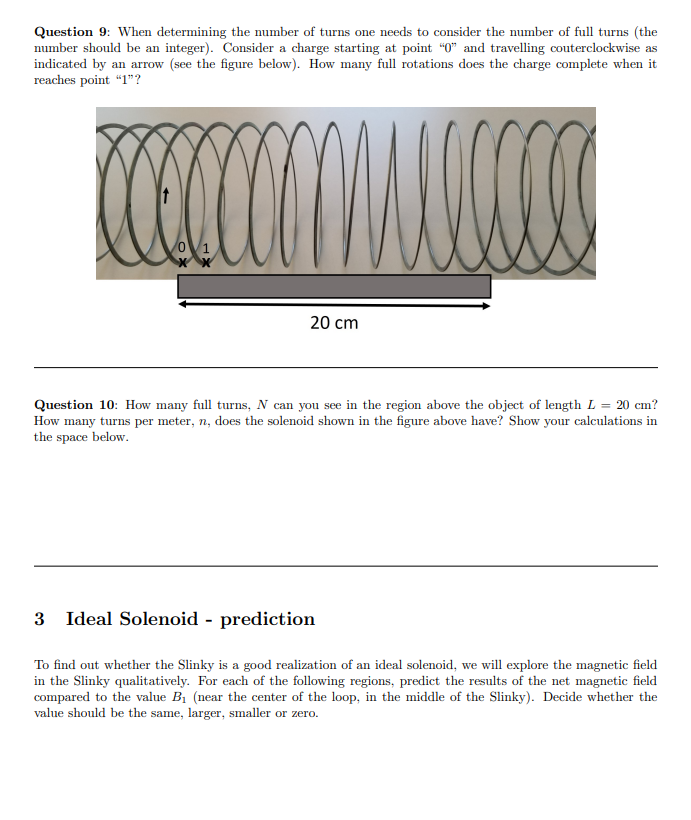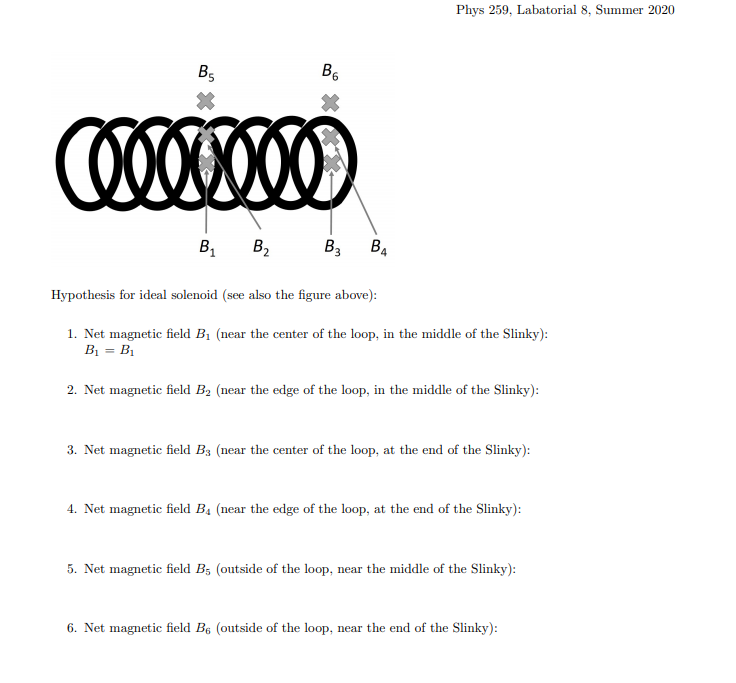For many technical applications, it is desirable to have a uniform magnetic field, i.e. a field with the same strength and direction at every point in a certain region of space. As you discovered in the last checkpoint, this can be achieved with a solenoid, which is constructed by "stacking" many loops of wire, each with the same current. The superposition of the magnetic fields of the single loops creates a strong, nearly uniform field inside the solenoid, whereas the field on the outside is weak and is approximately zero. 4 The magnetic field near the centre of an ideal solenoid is given by B = Honl, where Ho = 41 x 10-7 T-m/A is the magnetic constant, I is the current that runs through the solenoid, and n = N/L is the number of turns per unit length. Note that the magnetic field does not depend on the radius of the solenoid. Question 7: You are given a solenoid with n = 100 turns per meter and are asked to generate a magnetic field inside the solenoid that is large enough to cancel the magnetic field of the earth, the average value of which is 5 x 10-5 T on the surface of the earth. How big does the current have to be? Question 8: If you want this current to be as small as possible (for the magnetic field given in the previous question), should the solenoid be stretched or compressed? Answer this question by finding an algebraic expression for I in terms of n first, and then write your explanation.
Question 9: When determining the number of turns one needs to consider the number of full turns (the number should be an integer). Consider a charge starting at point "0" and travelling couterclockwise as indicated by an arrow (see the figure below). How many full rotations does the charge complete when it reaches point "1"? lom 20 cm Question 10: How many full turns, N can you see in the region above the object of length L = 20 cm? How many turns per meter, n, does the solenoid shown in the figure above have? Show your calculations in the space below. 3 Ideal Solenoid - prediction To find out whether the Slinky is a good realization of an ideal solenoid, we will explore the magnetic field in the Slinky qualitatively. For each of the following regions, predict the results of the net magnetic field compared to the value B (near the center of the loop, in the middle of the Slinky). Decide whether the value should be the same, larger, smaller or zero.
Phys 259, Labatorial 8, Summer 2020 B B6 Cooooooo B B2 B3 B4 Hypothesis for ideal solenoid (see also the figure above): 1. Net magnetic field B. (near the center of the loop, in the middle of the Slinky): B = B 2. Net magnetic field B2 (near the edge of the loop, in the middle of the Slinky): 3. Net magnetic field B3 (near the center of the loop, at the end of the Slinky): 4. Net magnetic field B. (near the edge of the loop, at the end of the Slinky): 5. Net magnetic field Bs (outside of the loop, near the middle of the Slinky): 6. Net magnetic field Be outside of the loop, near the end of the Slinky):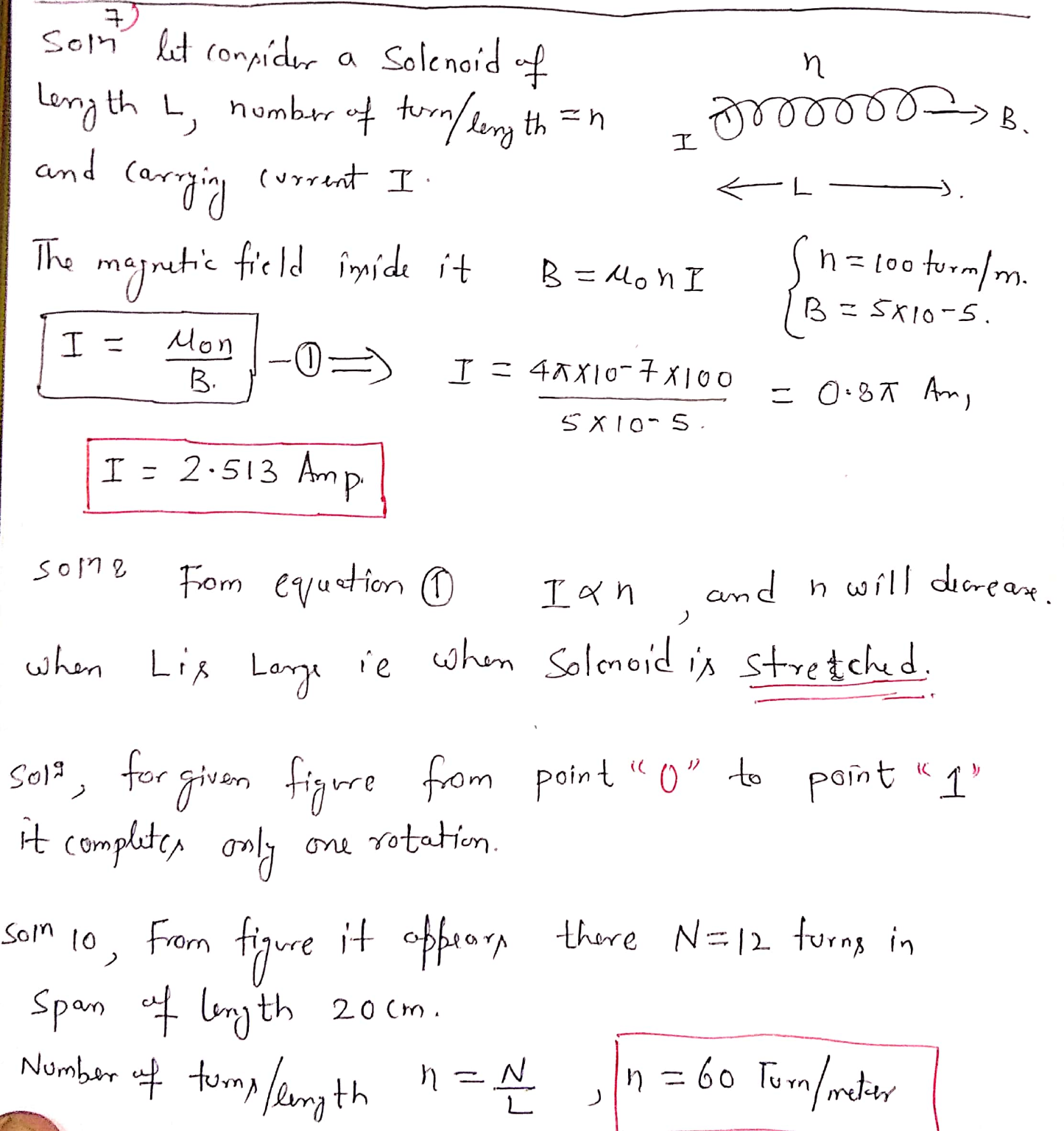##### Add Answer of: For many technical applications, it is desirable to have a uniform magnetic field, i.e. a field...
Similar Homework Help Questions
• ### 7 Ideal Solenoid - prediction To find out whether the Slinky is a good realization of...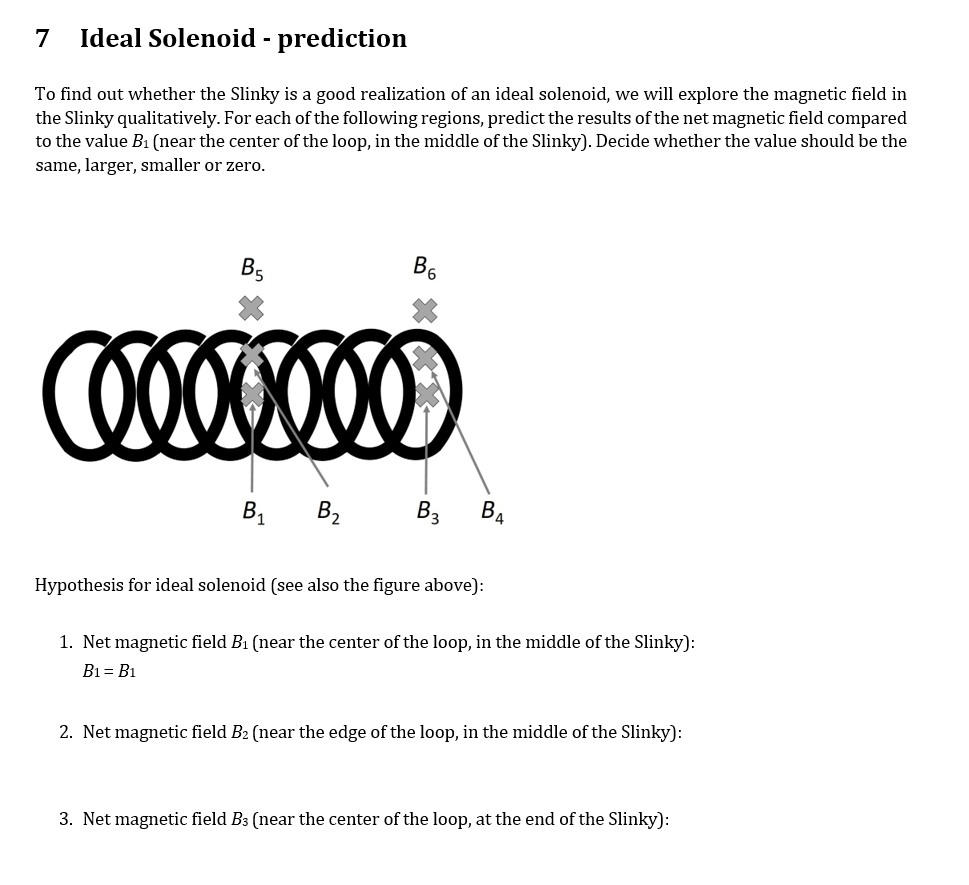7 Ideal Solenoid - prediction To find out whether the Slinky is a good realization of an ideal solenoid, we will explore the magnetic field in the Slinky qualitatively. For each of the following regions, predict the results of the net magnetic field compared to the value B1 (near the center of the loop, in the middle of the Slinky). Decide whether the value should be the same, larger, smaller or zero. B. B6 COOOO000 B. B2 B3 BA Hypothesis...

• ### Magnetic Field of a Coil

If have a loop of wire with one turn, and a current Iflowing through it then the magnetic field at the center of thecoil is given by:B=μ0I/2r,where μ0=1.2566x10-6N/A2 and r is the radius of the loop. When youhave N loops to form a coil, the magneticfield is justmultiplied by N:B=Nμ0I/2r.MAGNETIC FIELD OF A SOLENOID: If a coil is strected out toform a tube, then you have a solenoid. The magnetic field of asolenoid far from the ends is givenbyB=μ0In,where n...

• ### magnetic field of a solenoid

A solenoid has a radius of 4.00 cm and a length of 50.0 cm. The number density of the windings is 300 loops per meter. A current of 0.575 A flows through the solenoid.(a) What is the strength of the magnetic field inside the solenoid? (b) What is the strength of the magnetic field outside the solenoid, at a distance of 5.00 m fromits center? (c) What is the strength of the magnetic field inside the solenoid if its radius...

• ### 9.2 Derivation: magnetic field of a solenoid (Ampère)

A solenoid has a radius of 4.00 cm and a length of 50.0 cm. The number density of the windings is 300 loops per meter. A current of 0.575 A flows through the solenoid.(a) What is the strength of the magnetic field inside the solenoid? (b) What is the strength of the magnetic field outside the solenoid, at a distance of 5.00 m fromits center? (c) What is the strength of the magnetic field inside the solenoid if its radius...

• ### magnetic field and magnetic forces

A solenoid is designed to produce a 2.79×10-2 T magnetic field near its center. It has a radius of 1.40 cm and a length of 40.0 cm, and the wire carries a current of12.0 AA. How many turns must the solenoid have? ( N = ??? turns )B.What total length of wire is required to make this solenoid? ( length = ??? m)

• ### Magnetic FieldYou are asked to to place an object in a uniform magnetic field.Where do you place the object?A)Near the north pole of a magnet.B)Near the south pole of a magnet.C)Inside a long, current-carryingcoil.D)Near the center of a long, current-carryingwire.E)Near the center of a single current loop.Hint:The magnitudeof the field insidea tightly wound solenoid (away from the ends) with n = N/l turns per unitlength is B =m0nI. The direction of the field is given by the right-hand rule. Curl the...

• ### Magnetic Field

current-carrying wires are wrapped around the square solenoid of side a, length L, and n turns per meter. Assume the current runs clockwise (looking down on thecoil).a) At the geometric center of the cavity inside the solenoid: the magnetic fieldpoints away from you, into the pagepoints toward you, out of the pagepoints upwardpoints downwardpoints to the rightis approximately zeropoints to the left

• ### 4) With FlipltPhysics you have already found the field due to a circular loop of radius R carryin...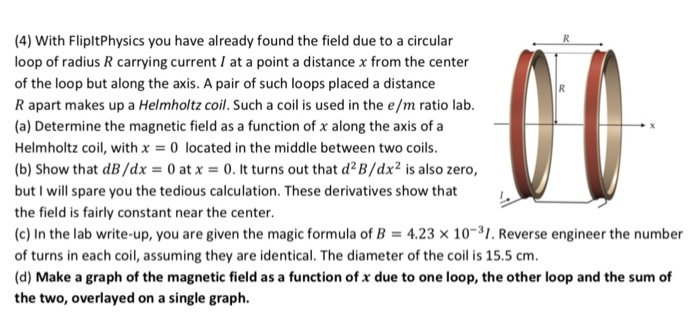4) With FlipltPhysics you have already found the field due to a circular loop of radius R carrying current I at a point a distance x from the center of the loop but along the axis. A pair of such loops placed a distance R apart makes up a Helmholtz coil. Such a coil is used in the e/m ratio lab (a) Determine the magnetic field as a function of x along the axis of a Helmholtz coil, with x...

• ### (4) With FlipltPhysics you have already found the field due to a circular loop of radius R carryi...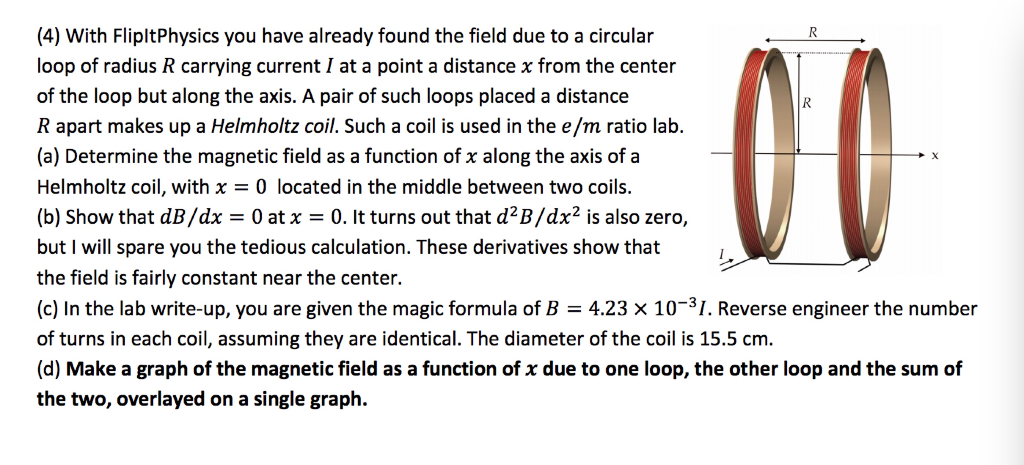(4) With FlipltPhysics you have already found the field due to a circular loop of radius R carrying current I at a point a distance x from the center of the loop but along the axis. A pair of such loops placed a distance R apart makes up a Helmholtz coil. Such a coil is used in the e/m ratio lab. (a) Determine the magnetic field as a function of x along the axis of a Helmholtz coil, with x...

• ### III. Extension to calculating the magnetic field on the axis of a solenoid . y! R...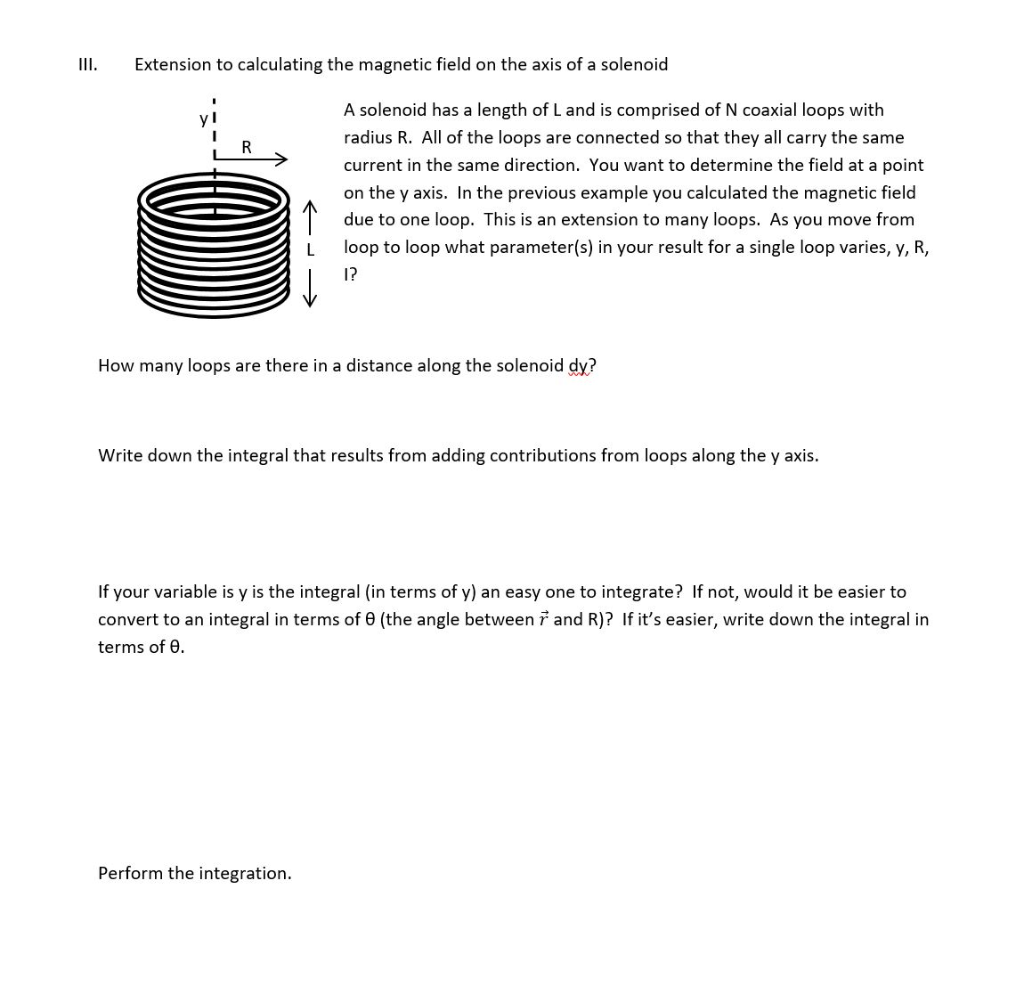III. Extension to calculating the magnetic field on the axis of a solenoid . y! R A solenoid has a length of Land is comprised of N coaxial loops with radius R. All of the loops are connected so that they all carry the same current in the same direction. You want to determine the field at a point on the y axis. In the previous example you calculated the magnetic field ^ due to one loop. This is an...

Free Homework App